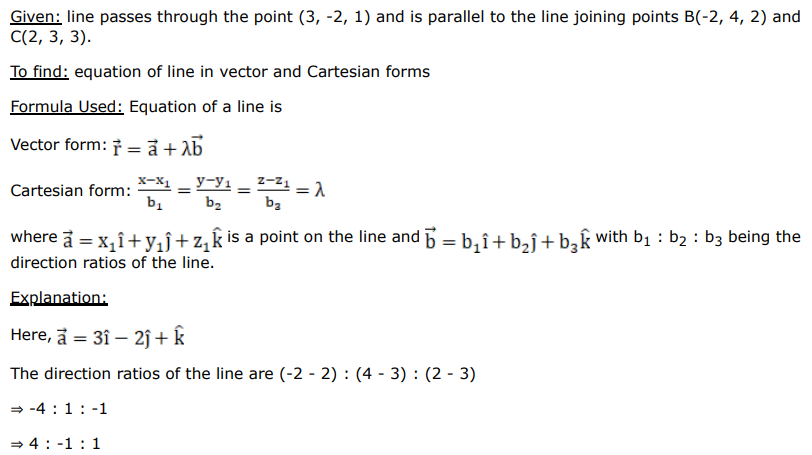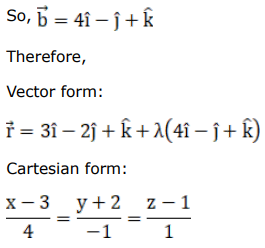# Find the vector equation of a line passing through the point

Question:

Find the vector equation of a line passing through the point $A(3,-2,1)$ and parallel to the line joining the points $B(-2,4,2)$ and $C(2,3,3)$. Also, find the Cartesian equations of the line.

Solution: